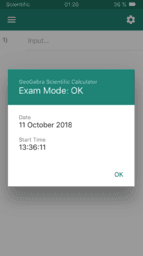# Learn GeoGebra Scientific Calculator

Welcome to the GeoGebra Scientific Calculator! Learn how to use the GeoGebra Scientific Calculator online and for Android and iOS mobile devices. Use the free GeoGebra Scientific Calculator for fractions, trigonometry and statistics.

# Learn GeoGebra Scientific Calculator

•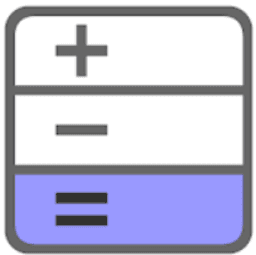### Introduction

•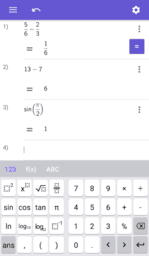### Explore the app

•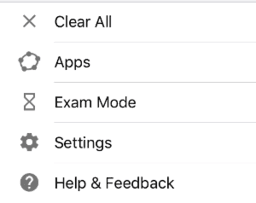•### Scientific Calculator Keyboard

•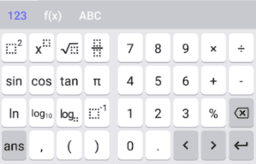### 123 Keyboard

•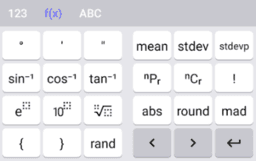### f(x) Keyboard

•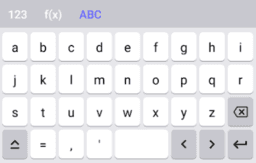### ABC Keyboard

•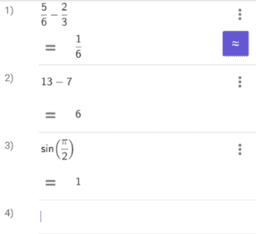### Input Bar

•### Predefined Functions and Operators

•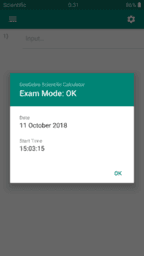### Android - Scientific Calculator Exam Mode

•